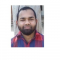## How to convert character column of a matrix into numeric in R?Nizamuddin Siddiqui

Updated on 22-Nov-2021 07:19:54

If we have a matrix that contains character columns and we want to convert a single column to numeric then we first need to convert the matrix into a data frame using as.data.frame function after that as.numeric function can be used to change the particular column to numeric type as ... Read More

## How to find the average of a particular column in R data frame?Nizamuddin Siddiqui

Updated on 22-Nov-2021 07:12:53

To find the average of a particular column in R data frame, we can take the help of delta (\$) operator.For example, if we have a data frame called df that contains a column x then we can find the average of column x by using the command given below ... Read More

## How to find the mean of all matrices stored in an R list?Nizamuddin Siddiqui

Updated on 22-Nov-2021 07:08:25

To find the mean of all matrices stored in an R list, we can use sapply function along with mean function. For example, if we have a list called LIST that contains some matrices then the mean of each matrix can be found by using the command given below −sapply(LIST, ... Read More

## How to update single value in an R data frame?Nizamuddin Siddiqui

Updated on 22-Nov-2021 07:04:37

To update single value in an R data frame, we can use row and column indices with single square brackets.For example, if we have a data frame called df that contains two columns and ten rows and if we want to change the fifth value in second column to 10 ... Read More

## How to find the coordinate of a value in an R matrix?Nizamuddin Siddiqui

Updated on 22-Nov-2021 07:00:27

The coordinate of a value in an R matrix is the row and column intersection that is the row and column index for that particular value. This can be found by using which function.For example, if we have a matrix called M that contains value starting from 1 to 20 ... Read More

## How to fill missing values after merging the data frames with another value than NA in R?Nizamuddin Siddiqui

Updated on 16-Nov-2021 07:12:20

To fill missing values after merging the data frames with another value than NA in R, we can follow the below steps −First of all, create two data frames.Then, merge the data frames by a common column between the two.After that, replace the NAs with another value.ExampleCreate the first data ... Read More

## How to use top_n function from dplyr to extract rows based on one column ordered in descending order in R?Nizamuddin Siddiqui

Updated on 16-Nov-2021 06:53:21

To use top_n function from dplyr to extract rows based on one column ordered indescending order in R, we can follow the below steps −First of all, create a data frame.Then, use top_n function dplyr package along with arrange and desc function to extract rows based on one column ordered ... Read More

## How to create quantile regression plot with larger width of lines using ggplot2 in R?Nizamuddin Siddiqui

Updated on 16-Nov-2021 06:48:20

To create quantile regression plot with larger width of lines using ggplot2 in R, we can follow the below steps −First of all, create a data frame.Then, use stat_quantile function with size argument and geom_point function of ggplot2 package to create quantile regression plot.ExampleCreate the data frameLet’s create a data ... Read More

## How to subset a matrix in R by specifying columns that contains NA?Nizamuddin Siddiqui

Updated on 16-Nov-2021 06:41:17

To subset a matrix in R by specifying columns that contains NA, we can follow the below steps −First of all, create a matrix with some NAs.Then, use is.na along with subset function to subset the matrix by specifying columns that contains NA.ExampleCreate the matrixLet’s create a matrix as shown ... Read More

## How to remove a row from matrix in R by using its name?Nizamuddin Siddiqui

Updated on 16-Nov-2021 06:33:30

To remove a row from matrix in R by using its name, we can follow the below steps −First of all, create a matrix.Then, add names to rows of the matrix.After that, subset the matrix by deselecting the desired row with negation and single square brackets for subsetting.ExampleCreate the matrixLet’s ... Read More